Скачать презентацию Planning Some material adopted from notes by Andreas

e92671dd7f6c4ccd6f7062cbe788d22e.ppt

• Количество слайдов: 45Planning Some material adopted from notes by Andreas Geyer-Schulz 1 and Chuck Dyer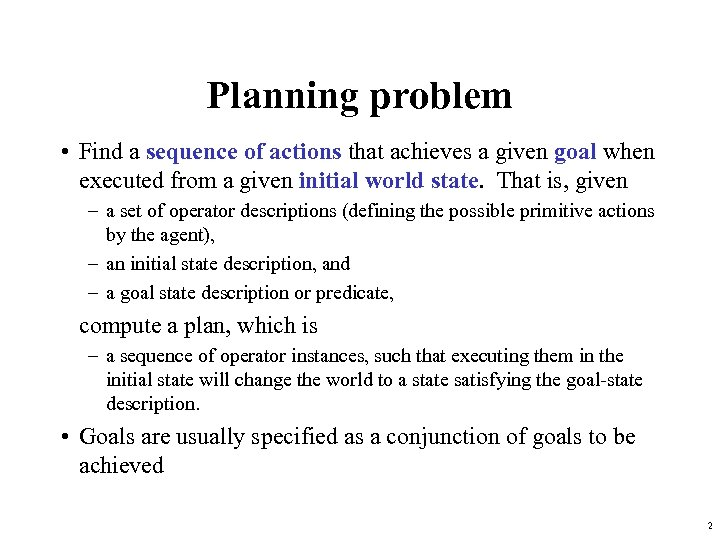Planning problem • Find a sequence of actions that achieves a given goal when executed from a given initial world state. That is, given – a set of operator descriptions (defining the possible primitive actions by the agent), – an initial state description, and – a goal state description or predicate, compute a plan, which is – a sequence of operator instances, such that executing them in the initial state will change the world to a state satisfying the goal-state description. • Goals are usually specified as a conjunction of goals to be achieved 2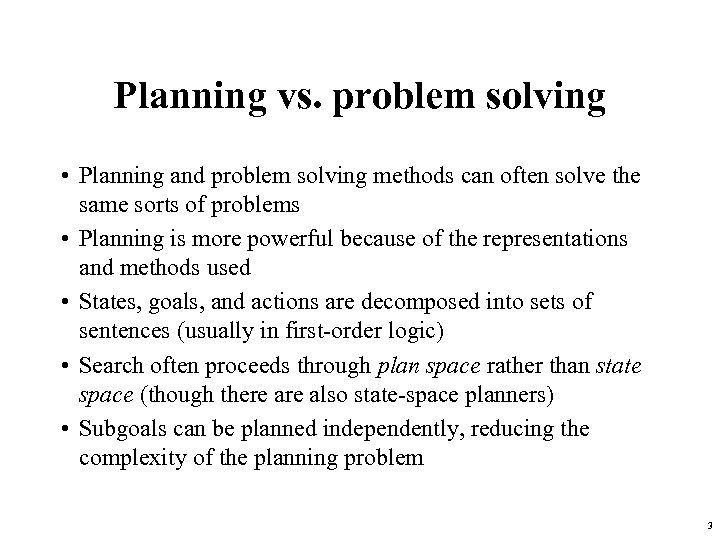Planning vs. problem solving • Planning and problem solving methods can often solve the same sorts of problems • Planning is more powerful because of the representations and methods used • States, goals, and actions are decomposed into sets of sentences (usually in first-order logic) • Search often proceeds through plan space rather than state space (though there also state-space planners) • Subgoals can be planned independently, reducing the complexity of the planning problem 3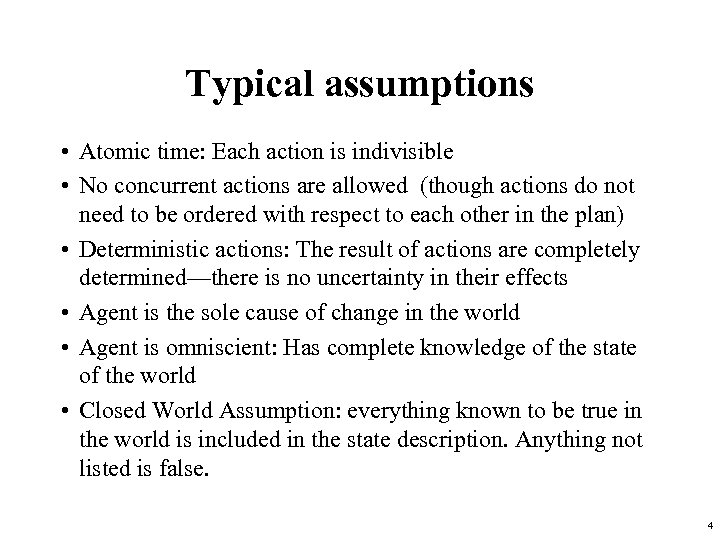Typical assumptions • Atomic time: Each action is indivisible • No concurrent actions are allowed (though actions do not need to be ordered with respect to each other in the plan) • Deterministic actions: The result of actions are completely determined—there is no uncertainty in their effects • Agent is the sole cause of change in the world • Agent is omniscient: Has complete knowledge of the state of the world • Closed World Assumption: everything known to be true in the world is included in the state description. Anything not listed is false. 4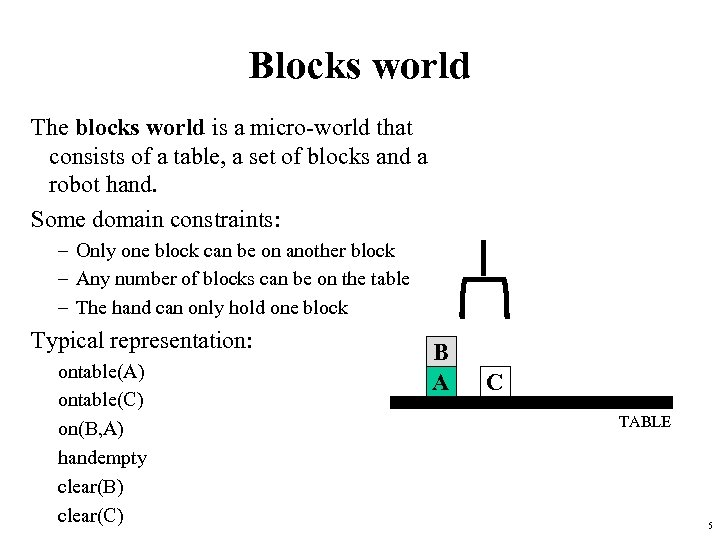Blocks world The blocks world is a micro-world that consists of a table, a set of blocks and a robot hand. Some domain constraints: – Only one block can be on another block – Any number of blocks can be on the table – The hand can only hold one block Typical representation: ontable(A) ontable(C) on(B, A) handempty clear(B) clear(C) B A C TABLE 5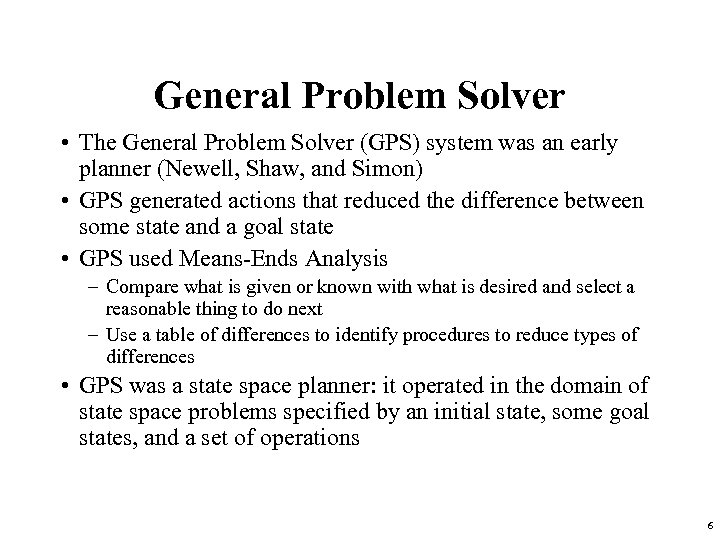General Problem Solver • The General Problem Solver (GPS) system was an early planner (Newell, Shaw, and Simon) • GPS generated actions that reduced the difference between some state and a goal state • GPS used Means-Ends Analysis – Compare what is given or known with what is desired and select a reasonable thing to do next – Use a table of differences to identify procedures to reduce types of differences • GPS was a state space planner: it operated in the domain of state space problems specified by an initial state, some goal states, and a set of operations 6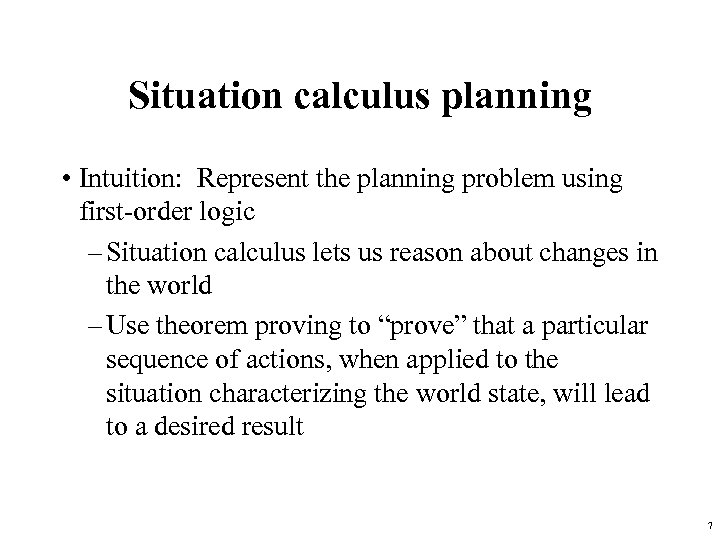Situation calculus planning • Intuition: Represent the planning problem using first-order logic – Situation calculus lets us reason about changes in the world – Use theorem proving to “prove” that a particular sequence of actions, when applied to the situation characterizing the world state, will lead to a desired result 7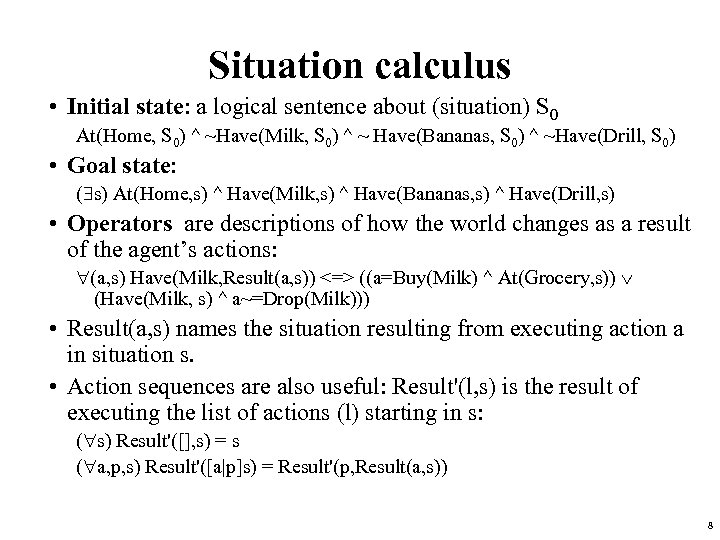Situation calculus • Initial state: a logical sentence about (situation) S 0 At(Home, S 0) ^ ~Have(Milk, S 0) ^ ~ Have(Bananas, S 0) ^ ~Have(Drill, S 0) • Goal state: ( s) At(Home, s) ^ Have(Milk, s) ^ Have(Bananas, s) ^ Have(Drill, s) • Operators are descriptions of how the world changes as a result of the agent’s actions: (a, s) Have(Milk, Result(a, s)) <=> ((a=Buy(Milk) ^ At(Grocery, s)) (Have(Milk, s) ^ a~=Drop(Milk))) • Result(a, s) names the situation resulting from executing action a in situation s. • Action sequences are also useful: Result'(l, s) is the result of executing the list of actions (l) starting in s: ( s) Result'([], s) = s ( a, p, s) Result'([a|p]s) = Result'(p, Result(a, s)) 8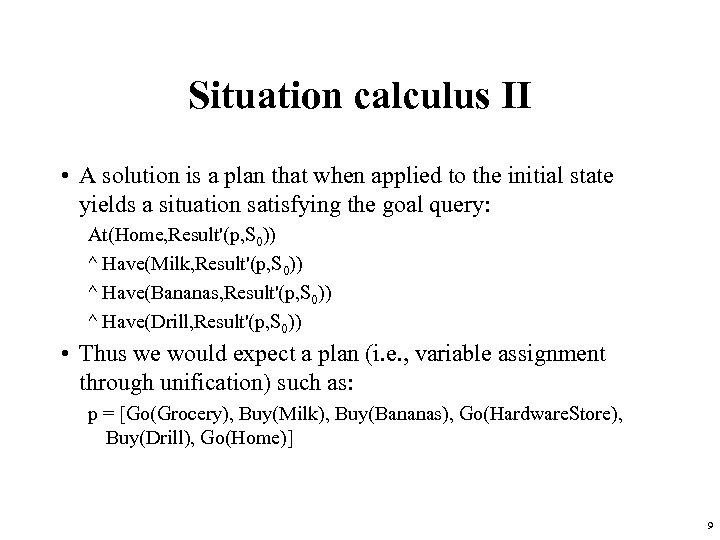Situation calculus II • A solution is a plan that when applied to the initial state yields a situation satisfying the goal query: At(Home, Result'(p, S 0)) ^ Have(Milk, Result'(p, S 0)) ^ Have(Bananas, Result'(p, S 0)) ^ Have(Drill, Result'(p, S 0)) • Thus we would expect a plan (i. e. , variable assignment through unification) such as: p = [Go(Grocery), Buy(Milk), Buy(Bananas), Go(Hardware. Store), Buy(Drill), Go(Home)] 9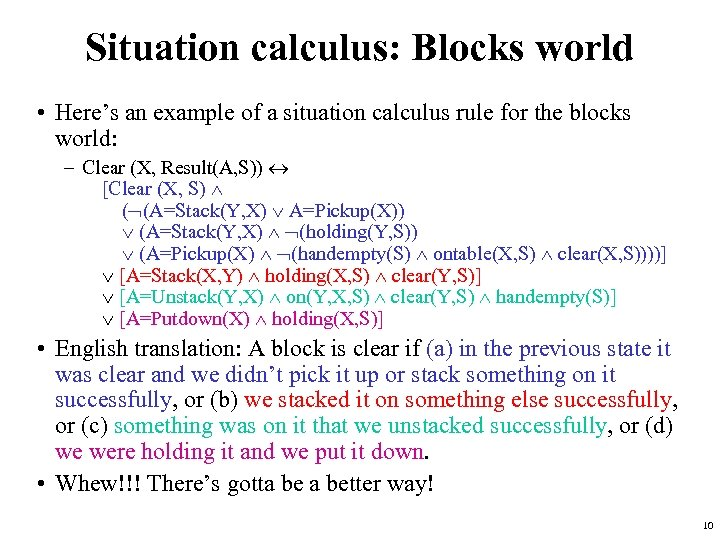Situation calculus: Blocks world • Here’s an example of a situation calculus rule for the blocks world: – Clear (X, Result(A, S)) [Clear (X, S) ( (A=Stack(Y, X) A=Pickup(X)) (A=Stack(Y, X) (holding(Y, S)) (A=Pickup(X) (handempty(S) ontable(X, S) clear(X, S))))] [A=Stack(X, Y) holding(X, S) clear(Y, S)] [A=Unstack(Y, X) on(Y, X, S) clear(Y, S) handempty(S)] [A=Putdown(X) holding(X, S)] • English translation: A block is clear if (a) in the previous state it was clear and we didn’t pick it up or stack something on it successfully, or (b) we stacked it on something else successfully, or (c) something was on it that we unstacked successfully, or (d) we were holding it and we put it down. • Whew!!! There’s gotta better way! 10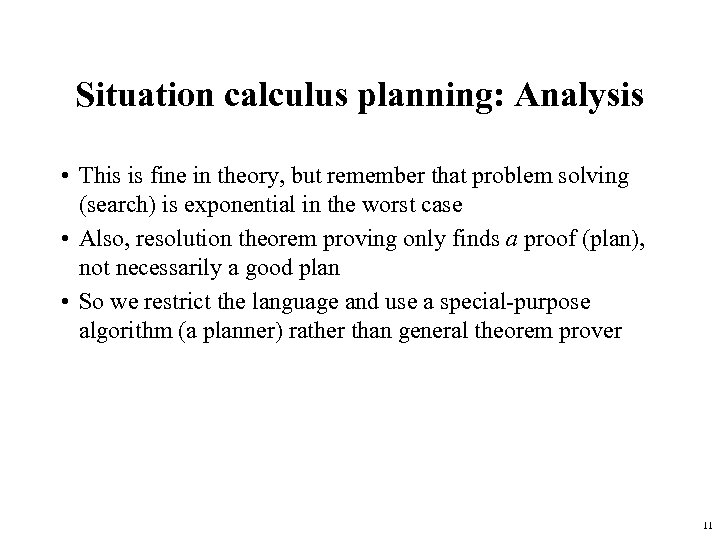Situation calculus planning: Analysis • This is fine in theory, but remember that problem solving (search) is exponential in the worst case • Also, resolution theorem proving only finds a proof (plan), not necessarily a good plan • So we restrict the language and use a special-purpose algorithm (a planner) rather than general theorem prover 11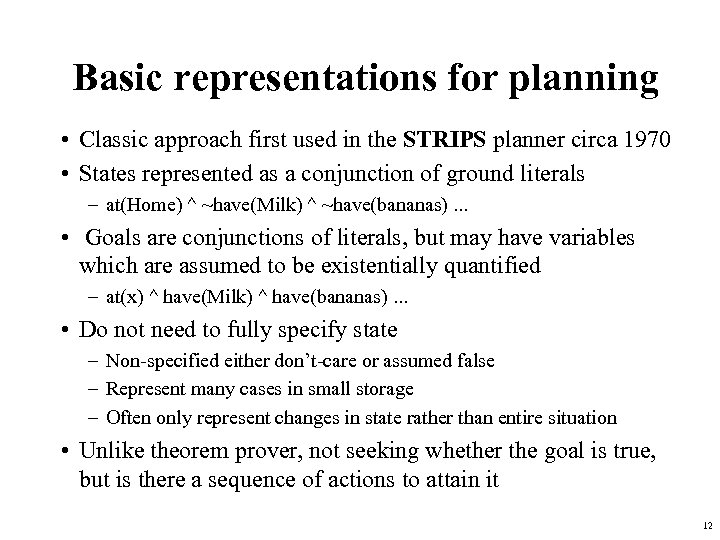Basic representations for planning • Classic approach first used in the STRIPS planner circa 1970 • States represented as a conjunction of ground literals – at(Home) ^ ~have(Milk) ^ ~have(bananas). . . • Goals are conjunctions of literals, but may have variables which are assumed to be existentially quantified – at(x) ^ have(Milk) ^ have(bananas). . . • Do not need to fully specify state – Non-specified either don’t-care or assumed false – Represent many cases in small storage – Often only represent changes in state rather than entire situation • Unlike theorem prover, not seeking whether the goal is true, but is there a sequence of actions to attain it 12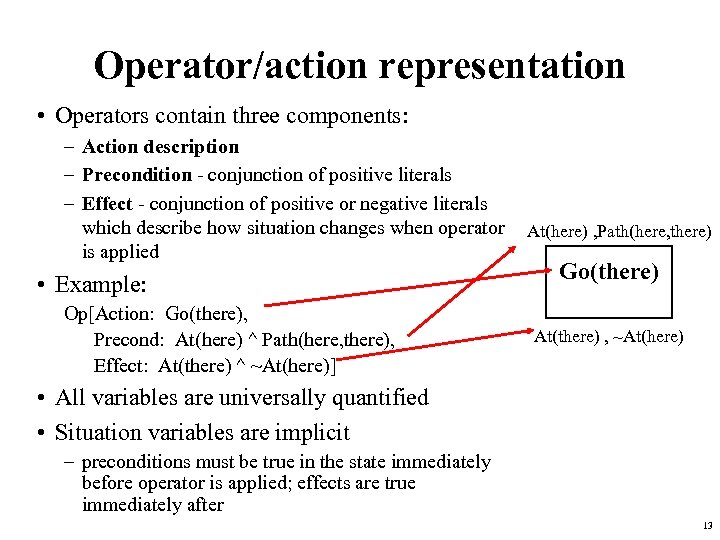Operator/action representation • Operators contain three components: – Action description – Precondition - conjunction of positive literals – Effect - conjunction of positive or negative literals which describe how situation changes when operator is applied • Example: Op[Action: Go(there), Precond: At(here) ^ Path(here, there), Effect: At(there) ^ ~At(here)] At(here) , Path(here, there) Go(there) At(there) , ~At(here) • All variables are universally quantified • Situation variables are implicit – preconditions must be true in the state immediately before operator is applied; effects are true immediately after 13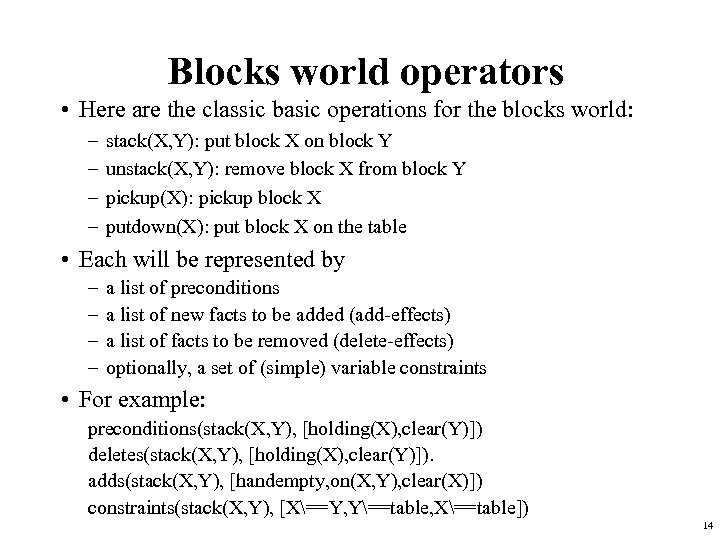Blocks world operators • Here are the classic basic operations for the blocks world: – – stack(X, Y): put block X on block Y unstack(X, Y): remove block X from block Y pickup(X): pickup block X putdown(X): put block X on the table • Each will be represented by – – a list of preconditions a list of new facts to be added (add-effects) a list of facts to be removed (delete-effects) optionally, a set of (simple) variable constraints • For example: preconditions(stack(X, Y), [holding(X), clear(Y)]) deletes(stack(X, Y), [holding(X), clear(Y)]). adds(stack(X, Y), [handempty, on(X, Y), clear(X)]) constraints(stack(X, Y), [X==Y, Y==table, X==table]) 14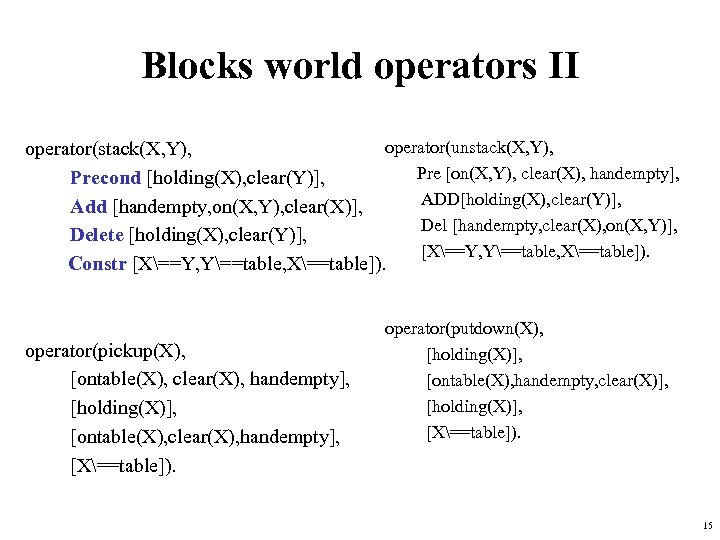Blocks world operators II operator(unstack(X, Y), operator(stack(X, Y), Pre [on(X, Y), clear(X), handempty], Precond [holding(X), clear(Y)], ADD[holding(X), clear(Y)], Add [handempty, on(X, Y), clear(X)], Del [handempty, clear(X), on(X, Y)], Delete [holding(X), clear(Y)], [X==Y, Y==table, X==table]). Constr [X==Y, Y==table, X==table]). operator(pickup(X), [ontable(X), clear(X), handempty], [holding(X)], [ontable(X), clear(X), handempty], [X==table]). operator(putdown(X), [holding(X)], [ontable(X), handempty, clear(X)], [holding(X)], [X==table]). 15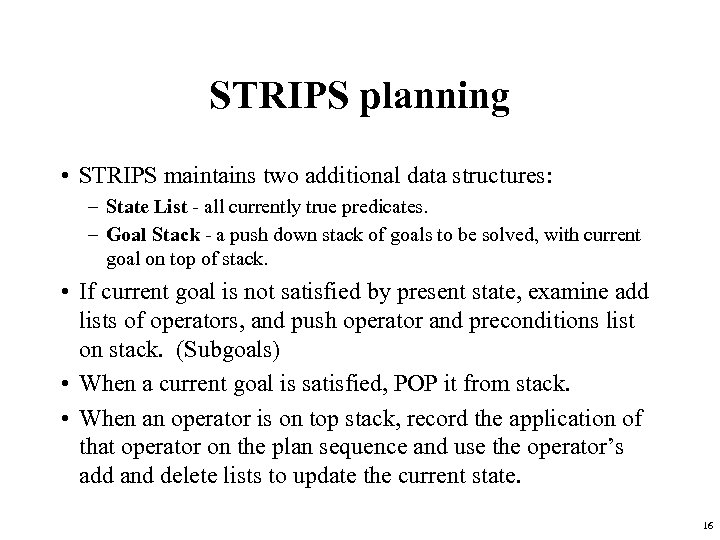STRIPS planning • STRIPS maintains two additional data structures: – State List - all currently true predicates. – Goal Stack - a push down stack of goals to be solved, with current goal on top of stack. • If current goal is not satisfied by present state, examine add lists of operators, and push operator and preconditions list on stack. (Subgoals) • When a current goal is satisfied, POP it from stack. • When an operator is on top stack, record the application of that operator on the plan sequence and use the operator’s add and delete lists to update the current state. 16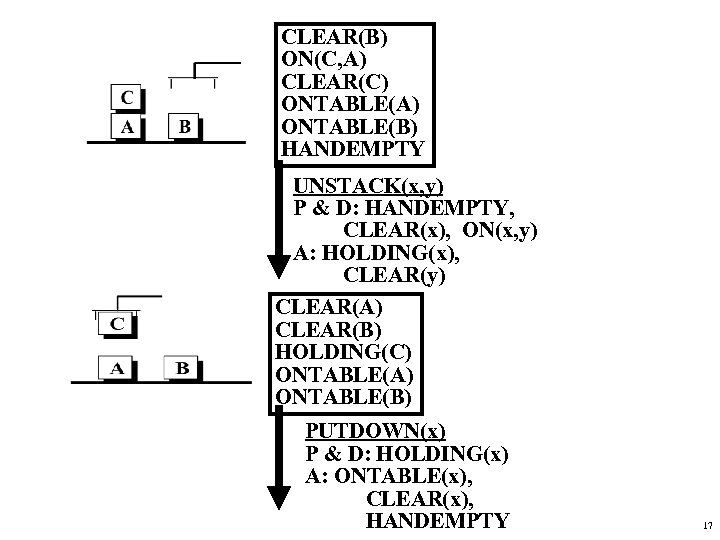CLEAR(B) ON(C, A) CLEAR(C) ONTABLE(A) ONTABLE(B) HANDEMPTY UNSTACK(x, y) P & D: HANDEMPTY, CLEAR(x), ON(x, y) A: HOLDING(x), CLEAR(y) CLEAR(A) CLEAR(B) HOLDING(C) ONTABLE(A) ONTABLE(B) PUTDOWN(x) P & D: HOLDING(x) A: ONTABLE(x), CLEAR(x), HANDEMPTY 17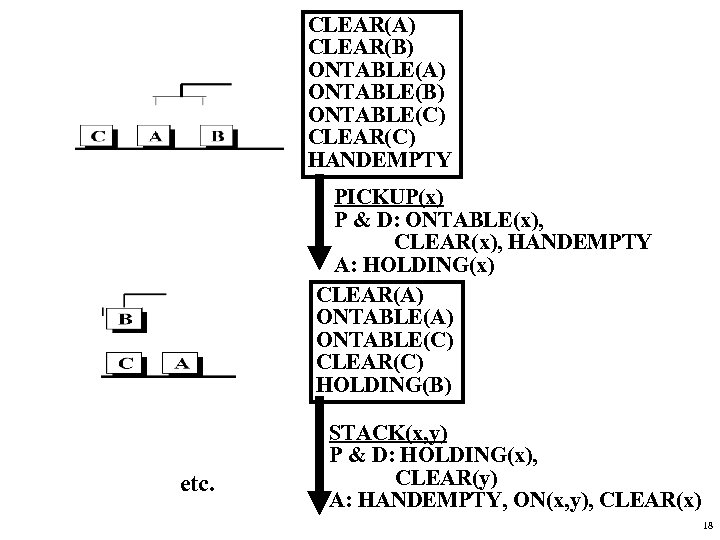CLEAR(A) CLEAR(B) ONTABLE(A) ONTABLE(B) ONTABLE(C) CLEAR(C) HANDEMPTY PICKUP(x) P & D: ONTABLE(x), CLEAR(x), HANDEMPTY A: HOLDING(x) CLEAR(A) ONTABLE(C) CLEAR(C) HOLDING(B) etc. STACK(x, y) P & D: HOLDING(x), CLEAR(y) A: HANDEMPTY, ON(x, y), CLEAR(x) 18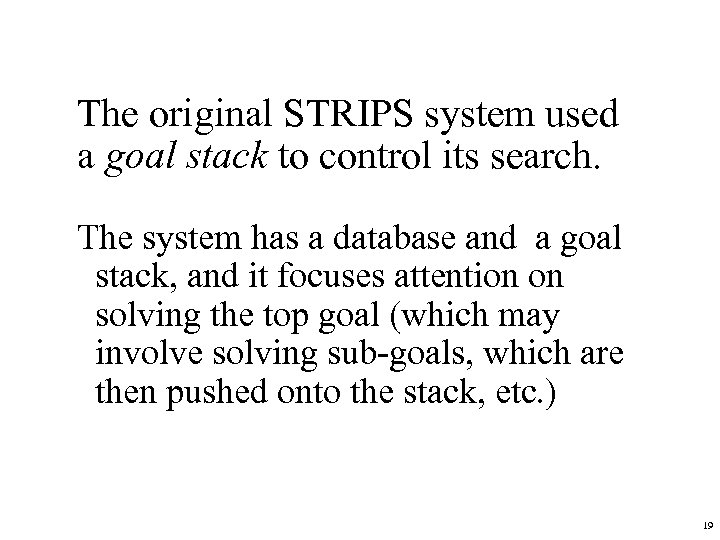The original STRIPS system used a goal stack to control its search. The system has a database and a goal stack, and it focuses attention on solving the top goal (which may involve solving sub-goals, which are then pushed onto the stack, etc. ) 19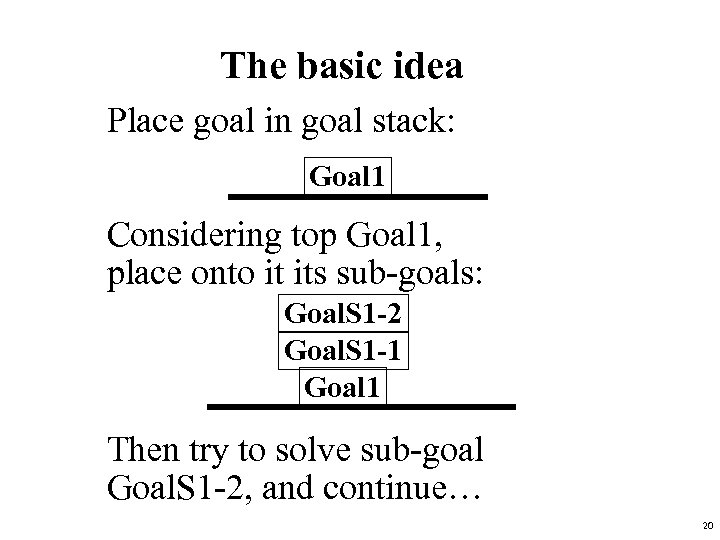The basic idea Place goal in goal stack: Goal 1 Considering top Goal 1, place onto it its sub-goals: Goal. S 1 -2 Goal. S 1 -1 Goal 1 Then try to solve sub-goal Goal. S 1 -2, and continue… 20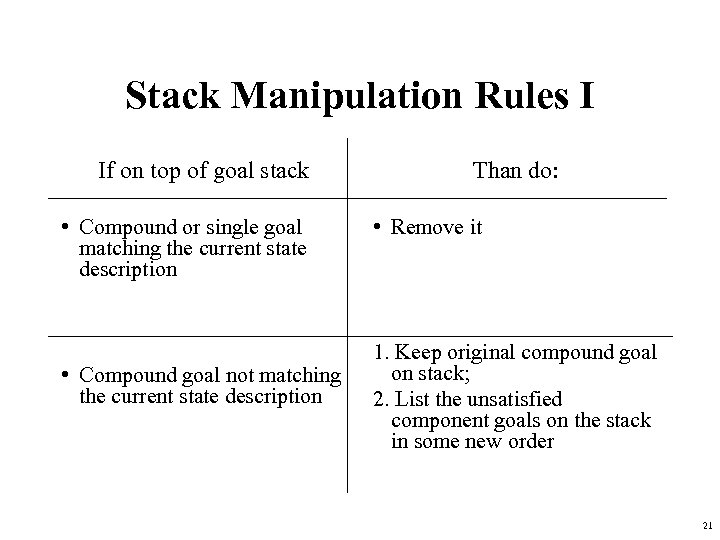Stack Manipulation Rules I If on top of goal stack • Compound or single goal matching the current state description • Compound goal not matching the current state description Than do: • Remove it 1. Keep original compound goal on stack; 2. List the unsatisfied component goals on the stack in some new order 21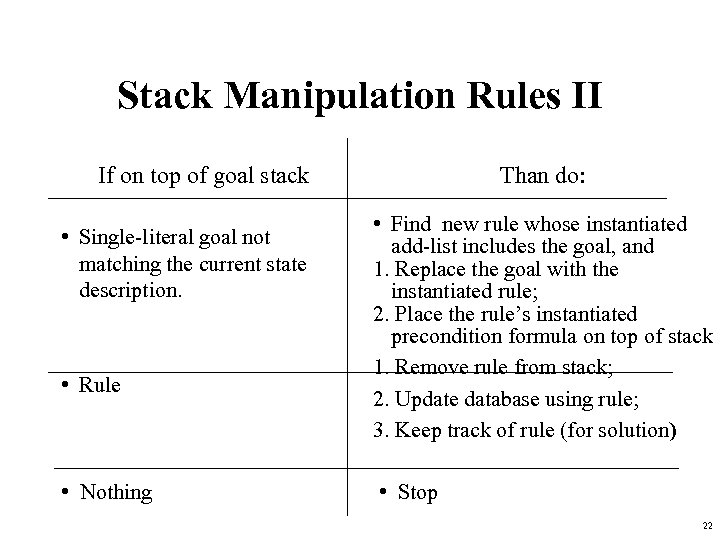Stack Manipulation Rules II If on top of goal stack • Single-literal goal not matching the current state description. • Rule • Nothing Than do: • Find new rule whose instantiated add-list includes the goal, and 1. Replace the goal with the instantiated rule; 2. Place the rule’s instantiated precondition formula on top of stack 1. Remove rule from stack; 2. Update database using rule; 3. Keep track of rule (for solution) • Stop 22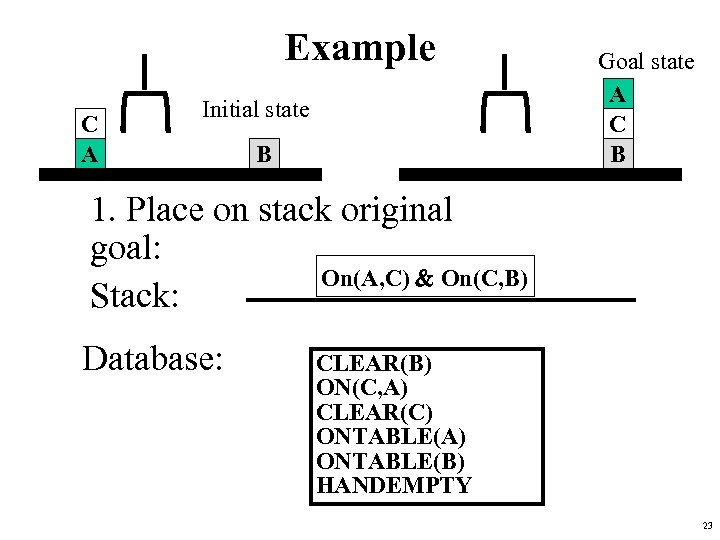Example C A Initial state B Goal state A C B 1. Place on stack original goal: On(A, C) & On(C, B) Stack: Database: CLEAR(B) ON(C, A) CLEAR(C) ONTABLE(A) ONTABLE(B) HANDEMPTY 23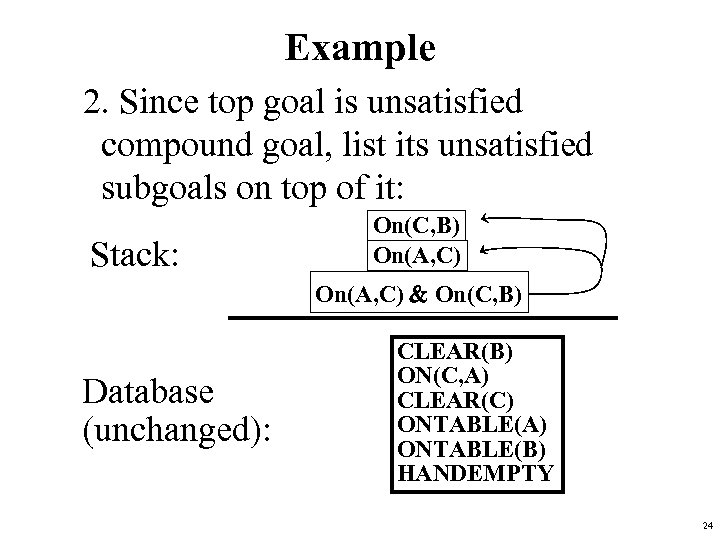Example 2. Since top goal is unsatisfied compound goal, list its unsatisfied subgoals on top of it: Stack: On(C, B) On(A, C) & On(C, B) Database (unchanged): CLEAR(B) ON(C, A) CLEAR(C) ONTABLE(A) ONTABLE(B) HANDEMPTY 24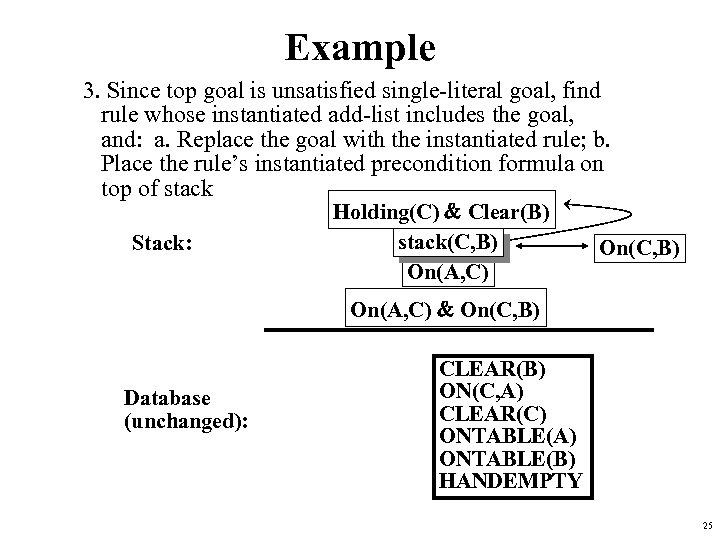Example 3. Since top goal is unsatisfied single-literal goal, find rule whose instantiated add-list includes the goal, and: a. Replace the goal with the instantiated rule; b. Place the rule’s instantiated precondition formula on top of stack Stack: Holding(C) & Clear(B) stack(C, B) On(A, C) On(C, B) On(A, C) & On(C, B) Database (unchanged): CLEAR(B) ON(C, A) CLEAR(C) ONTABLE(A) ONTABLE(B) HANDEMPTY 25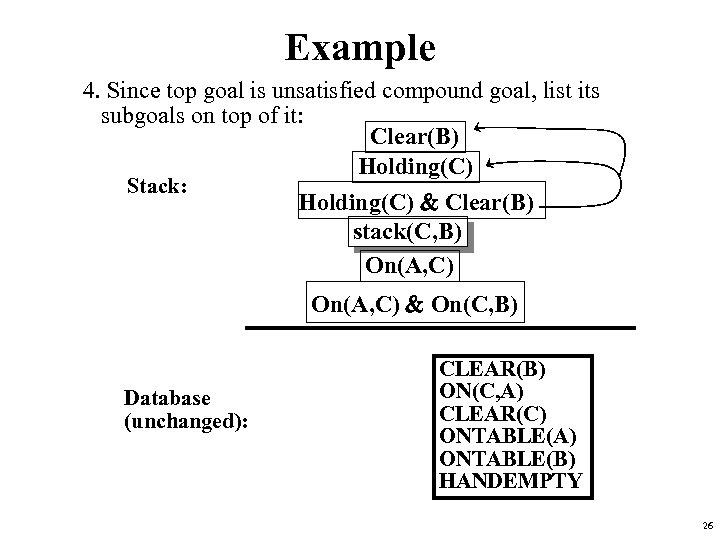Example 4. Since top goal is unsatisfied compound goal, list its subgoals on top of it: Clear(B) Holding(C) Stack: Holding(C) & Clear(B) stack(C, B) On(A, C) & On(C, B) Database (unchanged): CLEAR(B) ON(C, A) CLEAR(C) ONTABLE(A) ONTABLE(B) HANDEMPTY 26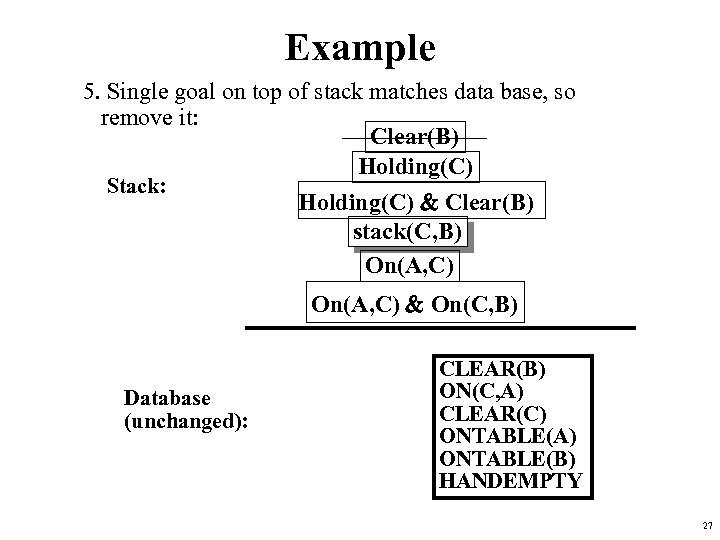Example 5. Single goal on top of stack matches data base, so remove it: Clear(B) Holding(C) Stack: Holding(C) & Clear(B) stack(C, B) On(A, C) & On(C, B) Database (unchanged): CLEAR(B) ON(C, A) CLEAR(C) ONTABLE(A) ONTABLE(B) HANDEMPTY 27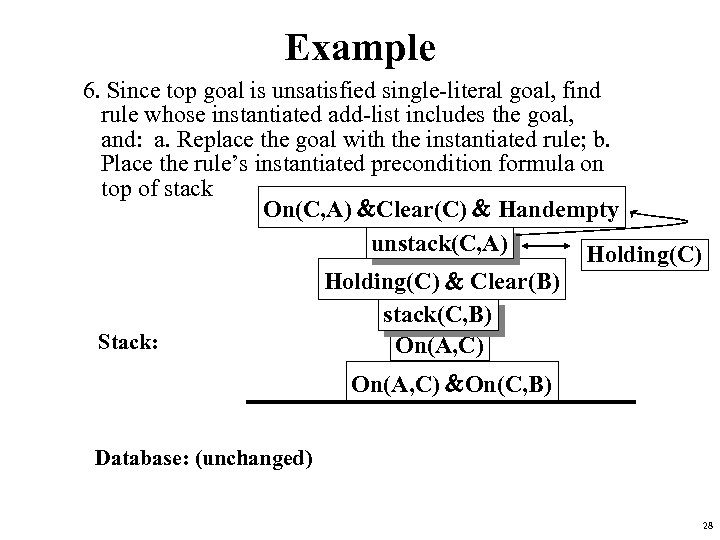Example 6. Since top goal is unsatisfied single-literal goal, find rule whose instantiated add-list includes the goal, and: a. Replace the goal with the instantiated rule; b. Place the rule’s instantiated precondition formula on top of stack On(C, A) &Clear(C) & Handempty unstack(C, A) Holding(C) Stack: Holding(C) & Clear(B) stack(C, B) On(A, C) &On(C, B) Database: (unchanged) 28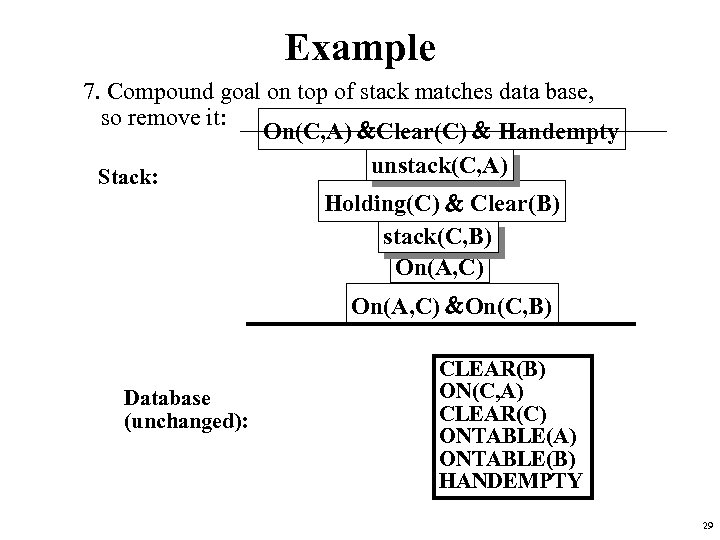Example 7. Compound goal on top of stack matches data base, so remove it: On(C, A) &Clear(C) & Handempty unstack(C, A) Stack: Holding(C) & Clear(B) stack(C, B) On(A, C) &On(C, B) Database (unchanged): CLEAR(B) ON(C, A) CLEAR(C) ONTABLE(A) ONTABLE(B) HANDEMPTY 29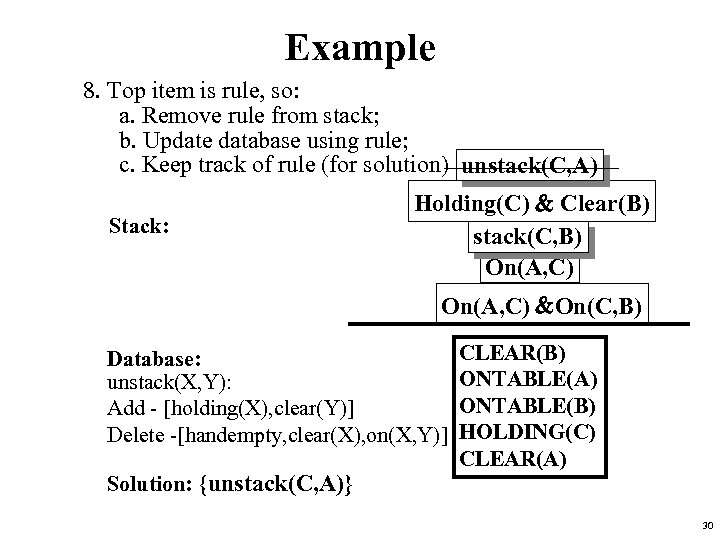Example 8. Top item is rule, so: a. Remove rule from stack; b. Update database using rule; c. Keep track of rule (for solution) unstack(C, A) Stack: Holding(C) & Clear(B) stack(C, B) On(A, C) &On(C, B) Database: unstack(X, Y): Add - [holding(X), clear(Y)] Delete -[handempty, clear(X), on(X, Y)] Solution: {unstack(C, A)} CLEAR(B) ONTABLE(A) ONTABLE(B) HOLDING(C) CLEAR(A) 30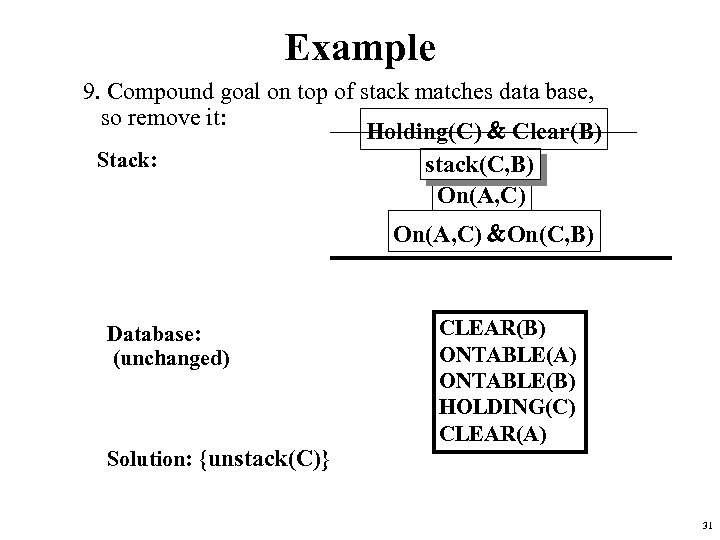Example 9. Compound goal on top of stack matches data base, so remove it: Holding(C) & Clear(B) Stack: stack(C, B) On(A, C) &On(C, B) Database: (unchanged) Solution: {unstack(C)} CLEAR(B) ONTABLE(A) ONTABLE(B) HOLDING(C) CLEAR(A) 31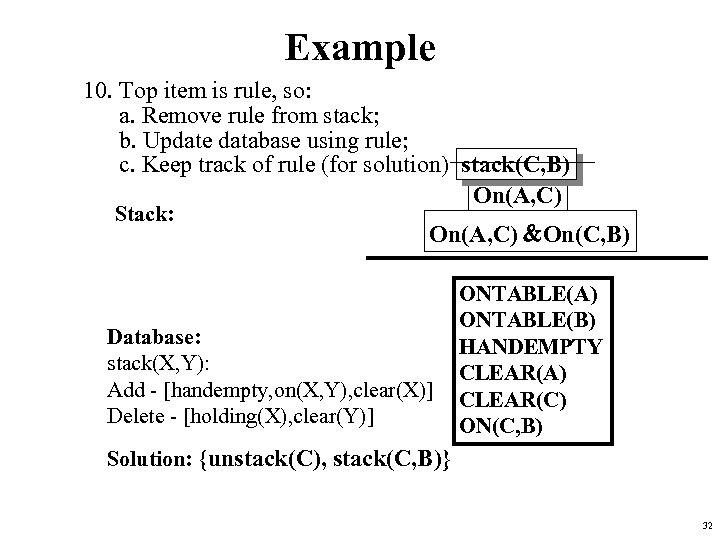Example 10. Top item is rule, so: a. Remove rule from stack; b. Update database using rule; c. Keep track of rule (for solution) stack(C, B) On(A, C) Stack: On(A, C) &On(C, B) Database: stack(X, Y): Add - [handempty, on(X, Y), clear(X)] Delete - [holding(X), clear(Y)] ONTABLE(A) ONTABLE(B) HANDEMPTY CLEAR(A) CLEAR(C) ON(C, B) Solution: {unstack(C), stack(C, B)} 32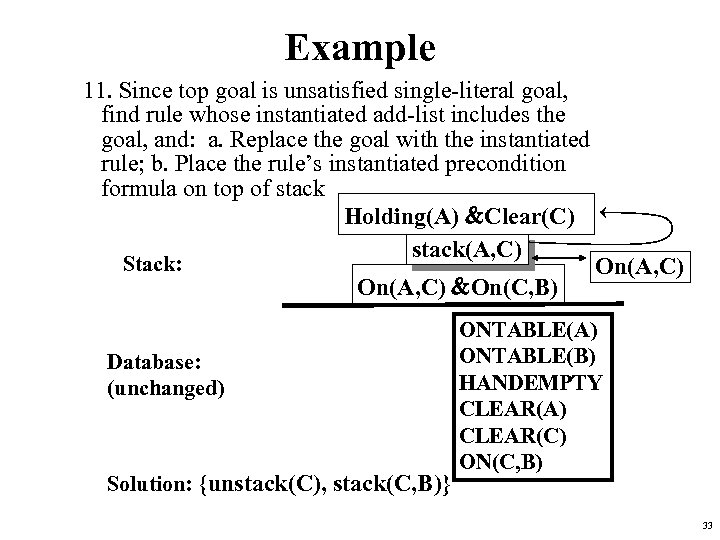Example 11. Since top goal is unsatisfied single-literal goal, find rule whose instantiated add-list includes the goal, and: a. Replace the goal with the instantiated rule; b. Place the rule’s instantiated precondition formula on top of stack Holding(A) &Clear(C) stack(A, C) Stack: On(A, C) &On(C, B) Database: (unchanged) Solution: {unstack(C), stack(C, B)} On(A, C) ONTABLE(A) ONTABLE(B) HANDEMPTY CLEAR(A) CLEAR(C) ON(C, B) 33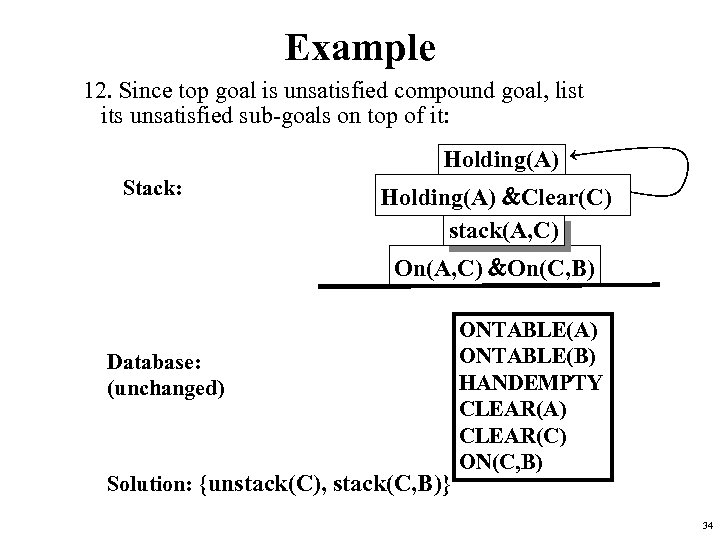Example 12. Since top goal is unsatisfied compound goal, list its unsatisfied sub-goals on top of it: Holding(A) Stack: Holding(A) &Clear(C) stack(A, C) On(A, C) &On(C, B) Database: (unchanged) Solution: {unstack(C), stack(C, B)} ONTABLE(A) ONTABLE(B) HANDEMPTY CLEAR(A) CLEAR(C) ON(C, B) 34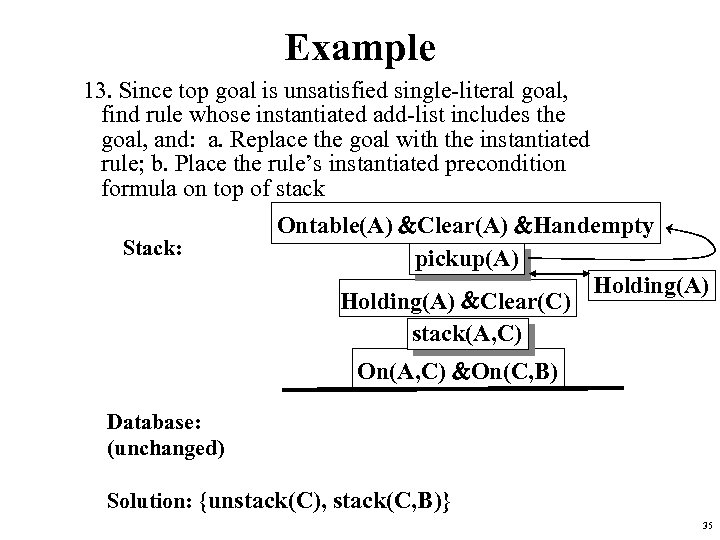Example 13. Since top goal is unsatisfied single-literal goal, find rule whose instantiated add-list includes the goal, and: a. Replace the goal with the instantiated rule; b. Place the rule’s instantiated precondition formula on top of stack Stack: Ontable(A) &Clear(A) &Handempty pickup(A) Holding(A) &Clear(C) stack(A, C) On(A, C) &On(C, B) Database: (unchanged) Solution: {unstack(C), stack(C, B)} 35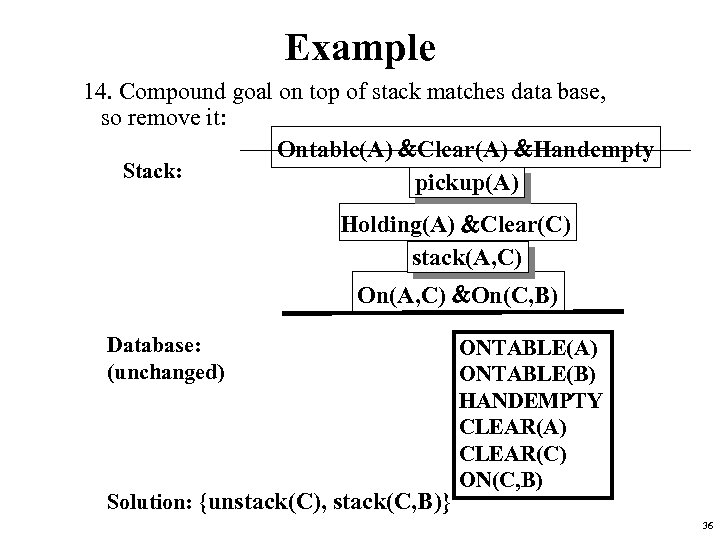Example 14. Compound goal on top of stack matches data base, so remove it: Ontable(A) &Clear(A) &Handempty Stack: pickup(A) Holding(A) &Clear(C) stack(A, C) On(A, C) &On(C, B) Database: (unchanged) Solution: {unstack(C), stack(C, B)} ONTABLE(A) ONTABLE(B) HANDEMPTY CLEAR(A) CLEAR(C) ON(C, B) 36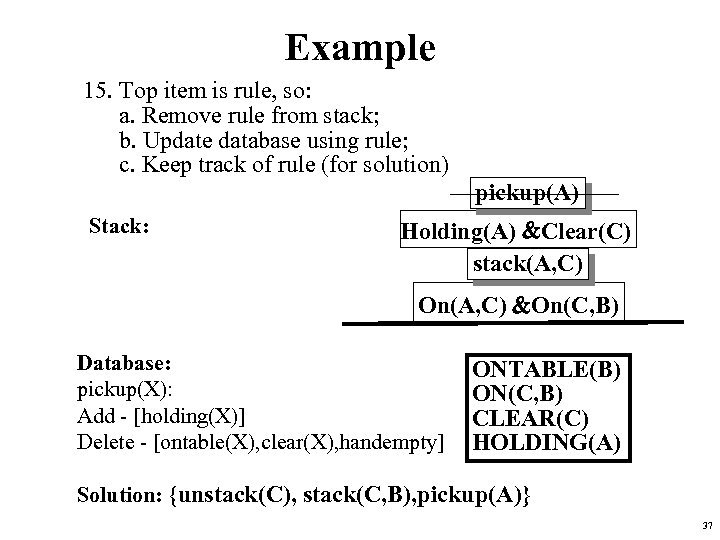Example 15. Top item is rule, so: a. Remove rule from stack; b. Update database using rule; c. Keep track of rule (for solution) pickup(A) Stack: Holding(A) &Clear(C) stack(A, C) On(A, C) &On(C, B) Database: pickup(X): Add - [holding(X)] Delete - [ontable(X), clear(X), handempty] ONTABLE(B) ON(C, B) CLEAR(C) HOLDING(A) Solution: {unstack(C), stack(C, B), pickup(A)} 37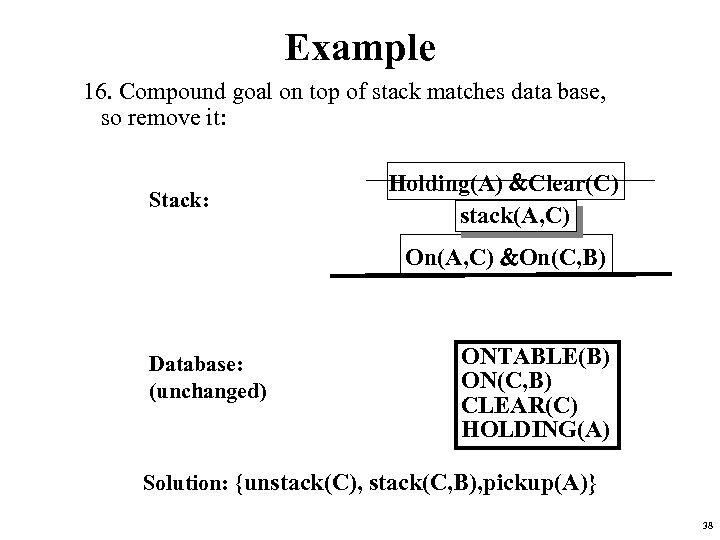Example 16. Compound goal on top of stack matches data base, so remove it: Stack: Holding(A) &Clear(C) stack(A, C) On(A, C) &On(C, B) Database: (unchanged) ONTABLE(B) ON(C, B) CLEAR(C) HOLDING(A) Solution: {unstack(C), stack(C, B), pickup(A)} 38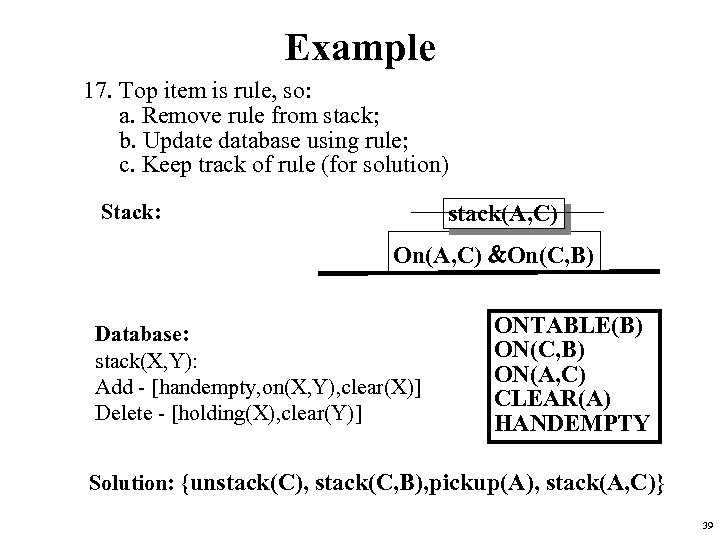Example 17. Top item is rule, so: a. Remove rule from stack; b. Update database using rule; c. Keep track of rule (for solution) Stack: stack(A, C) On(A, C) &On(C, B) Database: stack(X, Y): Add - [handempty, on(X, Y), clear(X)] Delete - [holding(X), clear(Y)] ONTABLE(B) ON(C, B) ON(A, C) CLEAR(A) HANDEMPTY Solution: {unstack(C), stack(C, B), pickup(A), stack(A, C)} 39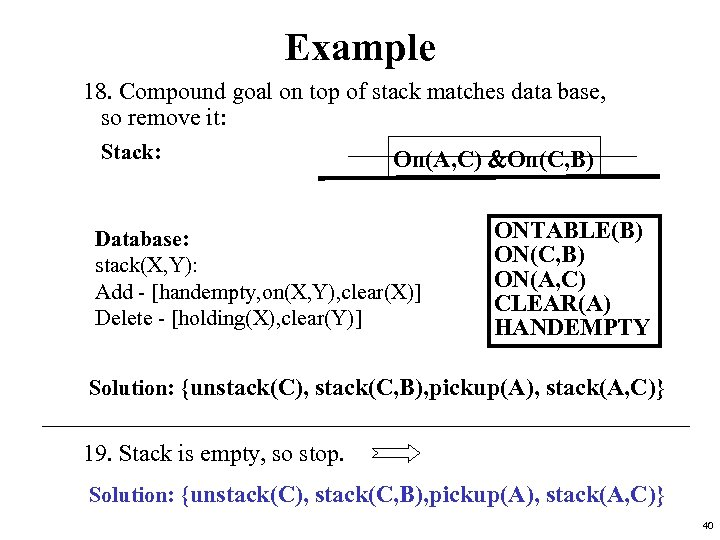Example 18. Compound goal on top of stack matches data base, so remove it: Stack: On(A, C) &On(C, B) Database: stack(X, Y): Add - [handempty, on(X, Y), clear(X)] Delete - [holding(X), clear(Y)] ONTABLE(B) ON(C, B) ON(A, C) CLEAR(A) HANDEMPTY Solution: {unstack(C), stack(C, B), pickup(A), stack(A, C)} 19. Stack is empty, so stop. Solution: {unstack(C), stack(C, B), pickup(A), stack(A, C)} 40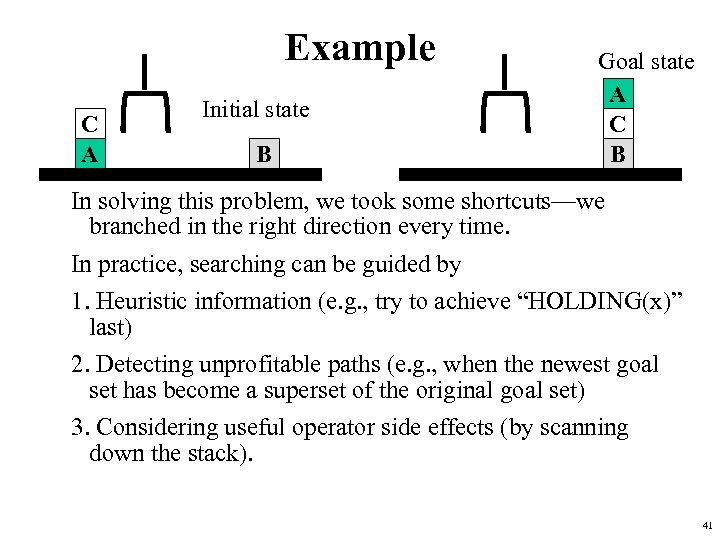Example C A Initial state B Goal state A C B In solving this problem, we took some shortcuts—we branched in the right direction every time. In practice, searching can be guided by 1. Heuristic information (e. g. , try to achieve “HOLDING(x)” last) 2. Detecting unprofitable paths (e. g. , when the newest goal set has become a superset of the original goal set) 3. Considering useful operator side effects (by scanning down the stack). 41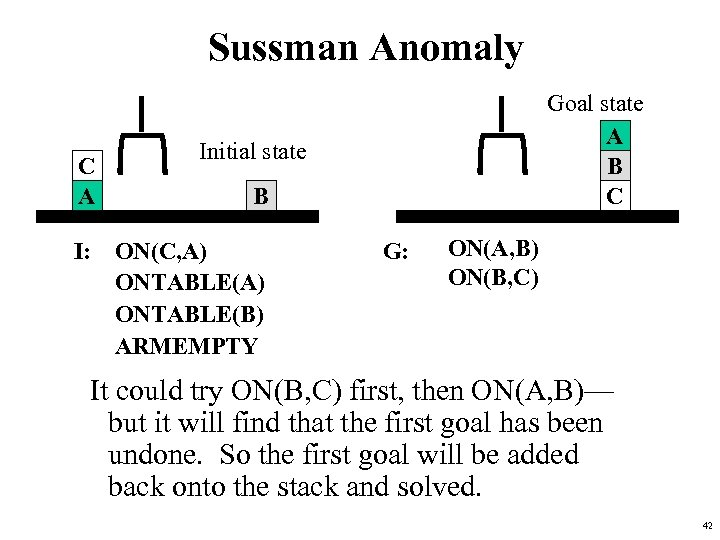Sussman Anomaly C A Goal state A B C Initial state B I: ON(C, A) ONTABLE(B) ARMEMPTY G: ON(A, B) ON(B, C) It could try ON(B, C) first, then ON(A, B)— but it will find that the first goal has been undone. So the first goal will be added back onto the stack and solved. 42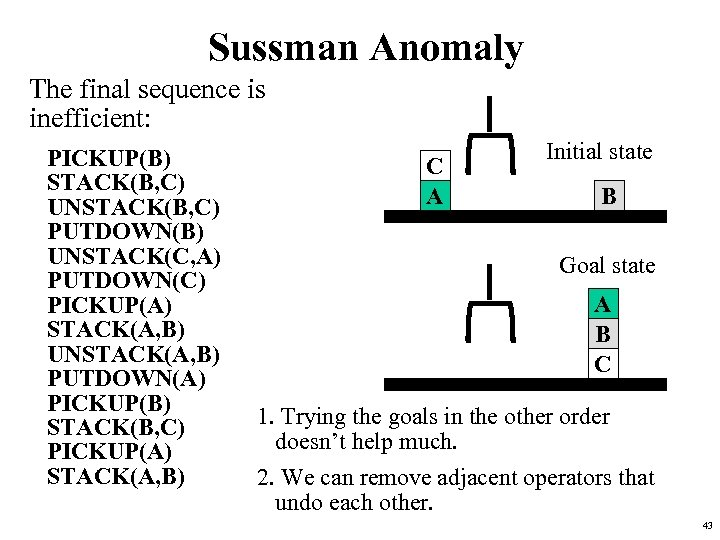Sussman Anomaly The final sequence is inefficient: PICKUP(B) STACK(B, C) UNSTACK(B, C) PUTDOWN(B) UNSTACK(C, A) PUTDOWN(C) PICKUP(A) STACK(A, B) UNSTACK(A, B) PUTDOWN(A) PICKUP(B) STACK(B, C) PICKUP(A) STACK(A, B) C A Initial state B Goal state A B C 1. Trying the goals in the other order doesn’t help much. 2. We can remove adjacent operators that undo each other. 43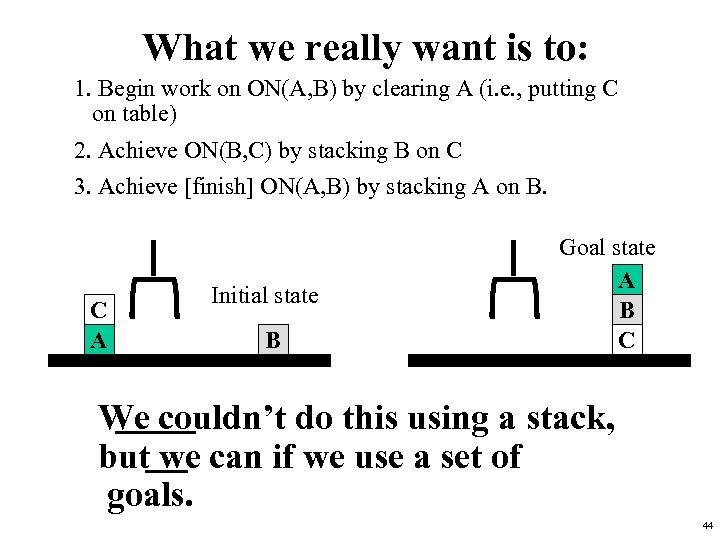What we really want is to: 1. Begin work on ON(A, B) by clearing A (i. e. , putting C on table) 2. Achieve ON(B, C) by stacking B on C 3. Achieve [finish] ON(A, B) by stacking A on B. C A Initial state B Goal state A B C We couldn’t do this using a stack, but we can if we use a set of goals. 44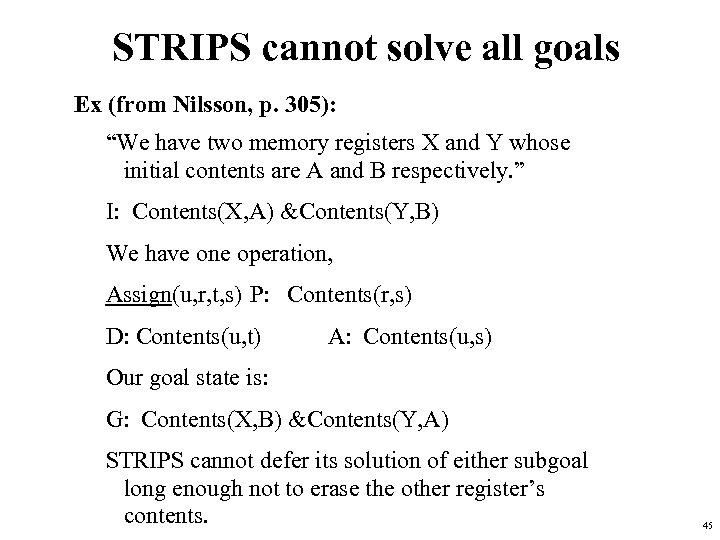STRIPS cannot solve all goals Ex (from Nilsson, p. 305): “We have two memory registers X and Y whose initial contents are A and B respectively. ” I: Contents(X, A) &Contents(Y, B) We have one operation, Assign(u, r, t, s) P: Contents(r, s) D: Contents(u, t) A: Contents(u, s) Our goal state is: G: Contents(X, B) &Contents(Y, A) STRIPS cannot defer its solution of either subgoal long enough not to erase the other register’s contents. 45